# LeNet

LeNet 即 LeNet5，由 Yann LeCun 在 1998 年提出，做为最早的卷积神经网络之一，是许多神经网络架构的起点。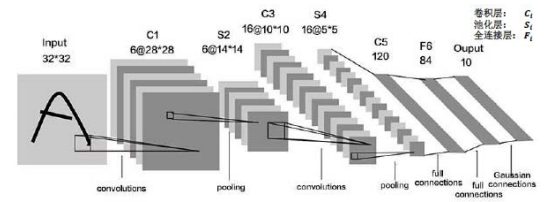C（6个5*5的卷积核，步长为1，不使用全零填充‘valid’）
B（不使用批标准化None）
A（使用Sigmoid激活函数）
P（使用最大池化max，池化核2*2，步长为2，不使用全零填充‘valid’）
D（None）

C（6个5*5的卷积核，步长为1，不使用全零填充‘valid’）
B（不使用批标准化None）
A（使用Sigmoid激活函数）
P（使用最大池化max，池化核2*2，步长为2，不使用全零填充‘valid’）
D（None）

Flatten（把卷积送过来的数据拉直）
Dense（神经元：120，激活函数：sigmoid）
Dense（神经元：84，激活函数：sigmoid）
Dense（神经元：10，激活函数：softmax）


LeNet源码

class LeNet5(Model):
def __init__(self):
super(LeNet5, self).__init__()
self.c1 = Conv2D(filters=6, kernel_size=(5, 5),
activation='sigmoid')
self.p1 = MaxPool2D(pool_size=(2, 2), strides=2)

self.c2 = Conv2D(filters=16, kernel_size=(5, 5),
activation='sigmoid')
self.p2 = MaxPool2D(pool_size=(2, 2), strides=2)

self.flatten = Flatten()
self.f1 = Dense(120, activation='sigmoid')
self.f2 = Dense(84, activation='sigmoid')
self.f3 = Dense(10, activation='softmax')


# AlexNet

AlexNet 的总体结构和 LeNet5 有相似之处。改进之处在于AlexNet共有8层，由五层卷积、三层全连接组成，输入图像尺寸为 224 * 224 * 3，网络规模远大于 LeNet5；使用 Relu 激活函数；进行了舍弃（Dropout）操作，以防止模型过拟合，提升鲁棒性；增加了一些训练上的技巧，包括数据增强、学习率衰减、权重衰减（L2 正则化）等。

AlexNet 的网络结构如图所示：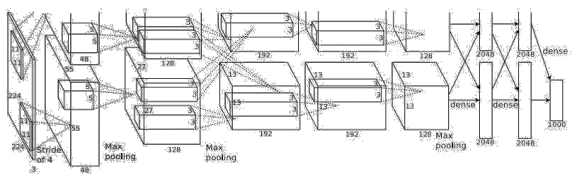Keras 实现 AlexNet 模型：

C（96个3*3的卷积核，步长为1，不使用全零填充‘valid’）
B（不使用批标准化Yes，‘LRN’）
A（使用rule激活函数）
P（使用最大池化max，池化核3*3，步长为2）
D（None）

C（256*3*3，步长为1，不使用全零填充‘valid’）
B（Yes，‘LRN’）
A（使用Relu激活函数）
P（max，3*3，步长为2）
D（None）

C（384*3*3，步长为1，全零填充‘same’）
B（None）
A（Relu）
P（None）
D（None）

C（384*3*3，步长为1，全零填充‘same’）
B（None）
A（Relu）
P（None）
D（None）

C（256*3*3，步长为1，全零填充‘same’）
B（None）
A（Relu）
P（max，核3*3，步长为2）
D（None）

Flatten
Dense（神经元：2048，激活函数：rule，Dropout：0.5）
Dense（神经元：2048，激活函数：rule，Dropout：0.5）
Dense（神经元：10，激活函数：softmax）


AlexNet源码

class AlexNet8(Model):
def __init__(self):
super(AlexNet8, self).__init__()
self.c1 = Conv2D(filters=96, kernel_size=(3, 3))
self.b1 = BatchNormalization()
self.a1 = Activation('relu')
self.p1 = MaxPool2D(pool_size=(3, 3), strides=2)

self.c2 = Conv2D(filters=256, kernel_size=(3, 3))
self.b2 = BatchNormalization()
self.a2 = Activation('relu')
self.p2 = MaxPool2D(pool_size=(3, 3), strides=2)

self.c3 = Conv2D(filters=384, kernel_size=(3, 3), padding='same',
activation='relu')

self.c4 = Conv2D(filters=384, kernel_size=(3, 3), padding='same',
activation='relu')

self.c5 = Conv2D(filters=256, kernel_size=(3, 3), padding='same',
activation='relu')
self.p3 = MaxPool2D(pool_size=(3, 3), strides=2)

self.flatten = Flatten()
self.f1 = Dense(2048, activation='relu')
self.d1 = Dropout(0.5)
self.f2 = Dense(2048, activation='relu')
self.d2 = Dropout(0.5)
self.f3 = Dense(10, activation='softmax')


# VGGNet

VGGNet 网络的最大改进是在网络的深度上，由 AlexNet 的 8 层增加到了 16 层和 19 层，更深的网络意味着更强的表达能力，这得益于强大的运算能力支持。VGGNet 的另一个显著特点是仅使用了单一尺寸的 3 * 3 卷积核，事实上，3 * 3 的小卷积核在很多卷积网络中都被大量使用，这是由于在感受野相同的情况下，小卷积核堆积的效果要优于大卷积核，同时参数量也更少。VGGNet 就使用了 3 * 3 的卷积核替代了 AlexNet 中的大卷积核（11 * 11、7 * 7、5 * 5），取得了较好的效果（事实上课程中利用 Keras 实现 AlexNet 时已经采取了这种方式）。

VGGNet16 和 VGGNet19 并没有本质上的区别，只是网络深度不同，前者 16 层（13 层卷积、3 层全连接），后者 19 层（16 层卷积、3 层全连接）。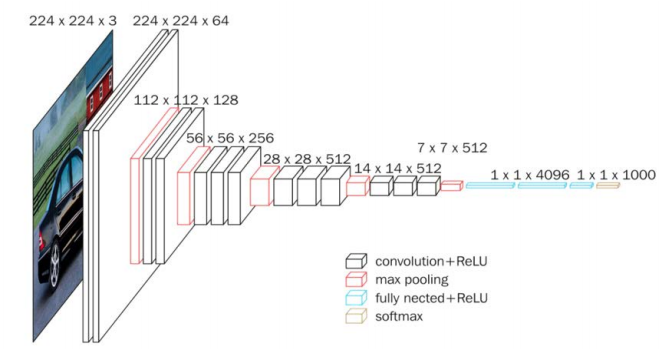C（核：64*3*3，步长：1，填充：same ）
B（Yes）A（relu）

C（核：64*3*3，步长：1，填充：same ）
B（Yes）A（relu）
P（max，核：2*2，步长：2） D（0.2）

C（核：128*3*3，步长：1，填充：same ）
B（Yes） A（relu）

C（核：128*3*3，步长：1，填充：same ）
B（Yes） A（relu）
P（max，核：2*2，步长：2） D（0.2）

C（核：256*3*3，步长：1，填充：same ）
B（Yes） A（relu）

C（核：256*3*3，步长：1，填充：same ）
B（Yes） A（relu）

C（核：256*3*3，步长：1，填充：same ）
B（Yes） A（relu）
P（max，核：2*2，步长：2） D（0.2）

C（核：512*3*3，步长：1，填充：same ）
B（Yes） A（relu）

C（核：512*3*3，步长：1，填充：same ）
B（Yes） A（relu）

C（核：512*3*3，步长：1，填充：same ）
B（Yes） A（relu）
P（max，核：2*2，步长：2） D（0.2）

C（核：512*3*3，步长：1，填充：same ）
B（Yes） A（relu）

C（核：512*3*3，步长：1，填充：same ）
B（Yes） A（relu）

C（核：512*3*3，步长：1，填充：same ）
B（Yes） A（relu）
P（max，核：2*2，步长：2） D（0.2）

Flatten
Dense（神经元：512，激活：relu，Dropout：0.2）
Dense（神经元：512，激活：relu，Dropout：0.2）
Dense（神经元：10，激活：softmax）


VGGNet源码

class VGG16(Model):
def __init__(self):
super(VGG16, self).__init__()
self.c1 = Conv2D(filters=64, kernel_size=(3, 3), padding='same')  # 卷积层1
self.b1 = BatchNormalization()  # BN层1
self.a1 = Activation('relu')  # 激活层1
self.c2 = Conv2D(filters=64, kernel_size=(3, 3), padding='same', )
self.b2 = BatchNormalization()  # BN层1
self.a2 = Activation('relu')  # 激活层1
self.p1 = MaxPool2D(pool_size=(2, 2), strides=2, padding='same')
self.d1 = Dropout(0.2)  # dropout层

self.c3 = Conv2D(filters=128, kernel_size=(3, 3), padding='same')
self.b3 = BatchNormalization()  # BN层1
self.a3 = Activation('relu')  # 激活层1
self.c4 = Conv2D(filters=128, kernel_size=(3, 3), padding='same')
self.b4 = BatchNormalization()  # BN层1
self.a4 = Activation('relu')  # 激活层1
self.p2 = MaxPool2D(pool_size=(2, 2), strides=2, padding='same')
self.d2 = Dropout(0.2)  # dropout层

self.c5 = Conv2D(filters=256, kernel_size=(3, 3), padding='same')
self.b5 = BatchNormalization()  # BN层1
self.a5 = Activation('relu')  # 激活层1
self.c6 = Conv2D(filters=256, kernel_size=(3, 3), padding='same')
self.b6 = BatchNormalization()  # BN层1
self.a6 = Activation('relu')  # 激活层1
self.c7 = Conv2D(filters=256, kernel_size=(3, 3), padding='same')
self.b7 = BatchNormalization()
self.a7 = Activation('relu')
self.p3 = MaxPool2D(pool_size=(2, 2), strides=2, padding='same')
self.d3 = Dropout(0.2)

self.c8 = Conv2D(filters=512, kernel_size=(3, 3), padding='same')
self.b8 = BatchNormalization()  # BN层1
self.a8 = Activation('relu')  # 激活层1
self.c9 = Conv2D(filters=512, kernel_size=(3, 3), padding='same')
self.b9 = BatchNormalization()  # BN层1
self.a9 = Activation('relu')  # 激活层1
self.c10 = Conv2D(filters=512, kernel_size=(3, 3), padding='same')
self.b10 = BatchNormalization()
self.a10 = Activation('relu')
self.p4 = MaxPool2D(pool_size=(2, 2), strides=2, padding='same')
self.d4 = Dropout(0.2)

self.c11 = Conv2D(filters=512, kernel_size=(3, 3), padding='same')
self.b11 = BatchNormalization()  # BN层1
self.a11 = Activation('relu')  # 激活层1
self.c12 = Conv2D(filters=512, kernel_size=(3, 3), padding='same')
self.b12 = BatchNormalization()  # BN层1
self.a12 = Activation('relu')  # 激活层1
self.c13 = Conv2D(filters=512, kernel_size=(3, 3), padding='same')
self.b13 = BatchNormalization()
self.a13 = Activation('relu')
self.p5 = MaxPool2D(pool_size=(2, 2), strides=2, padding='same')
self.d5 = Dropout(0.2)

self.flatten = Flatten()
self.f1 = Dense(512, activation='relu')
self.d6 = Dropout(0.2)
self.f2 = Dense(512, activation='relu')
self.d7 = Dropout(0.2)
self.f3 = Dense(10, activation='softmax')


# InceptionNet

InceptionNet 即 GoogLeNet，诞生于 2015 年，旨在通过增加网络的宽度来提升网络的能力，与 VGGNet 通过卷积层堆叠的方式（纵向）相比，是一个不同的方向（横向）。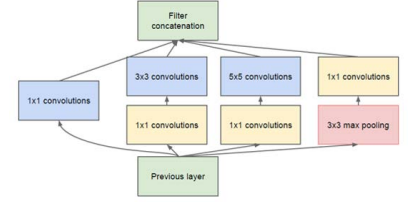InceptionNet引入了Inception结构块，在同一层网络内使用不同尺寸的卷积核，提升了模型的感知力，使用了批标准化，缓解了梯度消失。

分支一：
C（核：16*1*1，步长：1，填充：same ）
B（Yes） A（relu） P（None） D（None）

C（核：16*1*1，步长：1，填充：same ）
B（Yes） A（relu） P（None） D（None）

C（核：16*3*3，步长：1，填充：same ）
B（Yes） A（relu） P（None） D（None）

C（核：16*1*1，步长：1，填充：same ）
B（Yes） A（relu） P（None） D（None）

C（核：16*5*5，步长：1，填充：same ）
B（Yes） A（relu） P（None） D（None）

P（Max, 核：3*3， 步长：1 ，填充：same ）

C（核：16*1*1，步长：1，填充：same ）
B（Yes） A（relu） P（None） D（None）


InceptionNet源码

class ConvBNRelu(Model):
def __init__(self, ch, kernelsz=3, strides=1, padding='same'):
super(ConvBNRelu, self).__init__()
self.model = tf.keras.models.Sequential([
BatchNormalization(),
Activation('relu')
])

def call(self, x):
x = self.model(x, training=False)
return x


Inception结构块：

class InceptionBlk(Model):
def __init__(self, ch, strides=1):
super(InceptionBlk, self).__init__()
self.ch = ch
self.strides = strides
self.c1 = ConvBNRelu(ch, kernelsz=1, strides=strides)
self.c2_1 = ConvBNRelu(ch, kernelsz=1, strides=strides)
self.c2_2 = ConvBNRelu(ch, kernelsz=3, strides=1)
self.c3_1 = ConvBNRelu(ch, kernelsz=1, strides=strides)
self.c3_2 = ConvBNRelu(ch, kernelsz=5, strides=1)
self.c4_2 = ConvBNRelu(ch, kernelsz=1, strides=strides)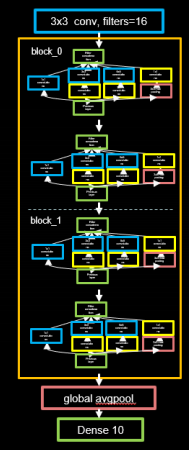class Inception10(Model):
def __init__(self, num_blocks, num_classes, init_ch=16, **kwargs):
super(Inception10, self).__init__(**kwargs)
self.in_channels = init_ch
self.out_channels = init_ch
self.num_blocks = num_blocks
self.init_ch = init_ch
self.c1 = ConvBNRelu(init_ch)
self.blocks = tf.keras.models.Sequential()
# InceptionNet 的基本单元，利用之前定义好的 InceptionBlk 类堆叠而成
for block_id in range(num_blocks):
for layer_id in range(2):
if layer_id == 0:
block = InceptionBlk(self.out_channels, strides=2)
else:
block = InceptionBlk(self.out_channels, strides=1)
# enlarger out_channels per block
self.out_channels *= 2
self.p1 = GlobalAveragePooling2D()
self.f1 = Dense(num_classes, activation='softmax')


InceptionNet 网络不再像 VGGNet 一样有三层全连接层（全连接层的参数量占 VGGNet 总参数量的 90 %），而是采用“全局平均池化+全连接层”的方式，这减少了大量的参数。

# ResNet

ResNet 即深度残差网络，由何恺明及其团队提出，是深度学习领域又一具有开创性的工作，通过对残差结构的运用，ResNet 使得训练数百层的网络成为了可能，从而具有非常强大的表征能力。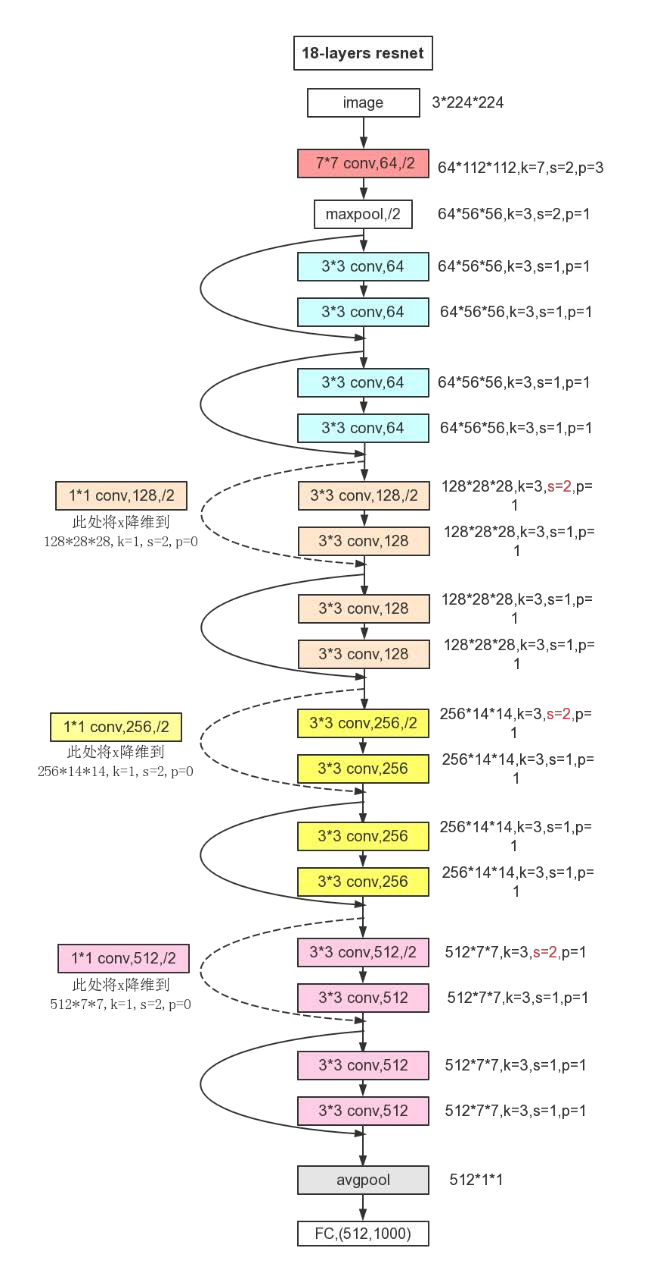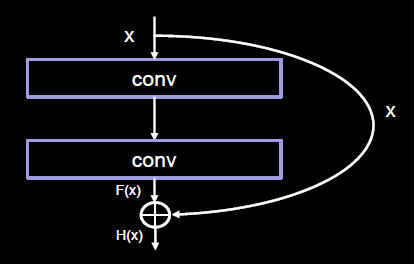ResNet 引入残差结构最主要的目的是解决网络层数不断加深时导致的梯度消失问题，从之前介绍的 4 种 CNN 经典网络结构我们也可以看出，网络层数的发展趋势是不断加深的。这是由于深度网络本身集成了低层/中层/高层特征和分类器，以多层首尾相连的方式存在，所以可以通过增加堆叠的层数（深度）来丰富特征的层次，以取得更好的效果。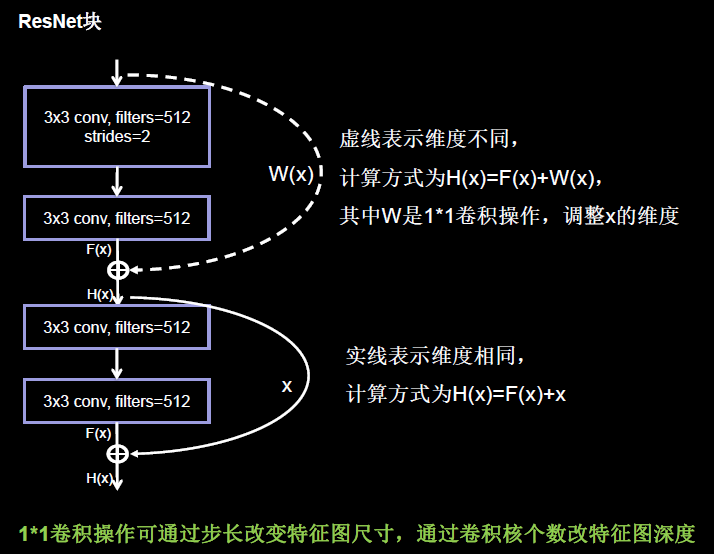class ResnetBlock(Model):

def __init__(self, filters, strides=1, residual_path=False):
super(ResnetBlock, self).__init__()
self.filters = filters
self.strides = strides
self.residual_path = residual_path

self.c1 = Conv2D(filters, (3, 3), strides=strides, padding='same', use_bias=False)
self.b1 = BatchNormalization()
self.a1 = Activation('relu')

self.c2 = Conv2D(filters, (3, 3), strides=1, padding='same', use_bias=False)
self.b2 = BatchNormalization()

# residual_path为True时，对输入进行下采样，即用1x1的卷积核做卷积操作，保证x能和F(x)维度相同，顺利相加
if residual_path:
self.down_c1 = Conv2D(filters, (1, 1), strides=strides, padding='same', use_bias=False)
self.down_b1 = BatchNormalization()

self.a2 = Activation('relu')

class ResNet18(Model):
def __init__(self, block_list, initial_filters=64):  # block_list表示每个block有几个卷积层
super(ResNet18, self).__init__()
self.num_blocks = len(block_list)  # 共有几个block
self.block_list = block_list
self.out_filters = initial_filters
self.c1 = Conv2D(self.out_filters, (3, 3), strides=1, padding='same', use_bias=False)
self.b1 = BatchNormalization()
self.a1 = Activation('relu')
self.blocks = tf.keras.models.Sequential()
# 构建ResNet网络结构
for block_id in range(len(block_list)):  # 第几个resnet block
for layer_id in range(block_list[block_id]):  # 第几个卷积层

if block_id != 0 and layer_id == 0:  # 对除第一个block以外的每个block的输入进行下采样
block = ResnetBlock(self.out_filters, strides=2, residual_path=True)
else:
block = ResnetBlock(self.out_filters, residual_path=False)
self.out_filters *= 2  # 下一个block的卷积核数是上一个block的2倍
self.p1 = tf.keras.layers.GlobalAveragePooling2D()
self.f1 = tf.keras.layers.Dense(10, activation='softmax', kernel_regularizer=tf.keras.regularizers.l2())


# 小结

LeNet5：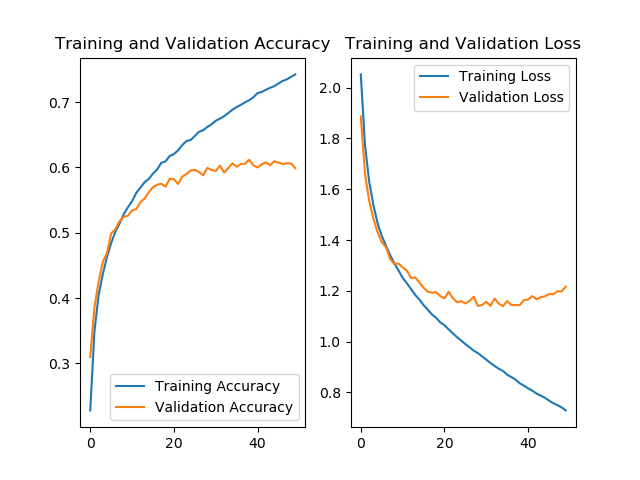AlexNet8：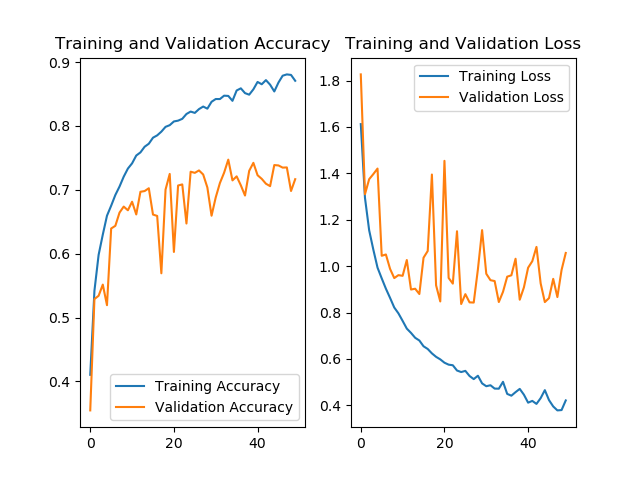VGGNet16：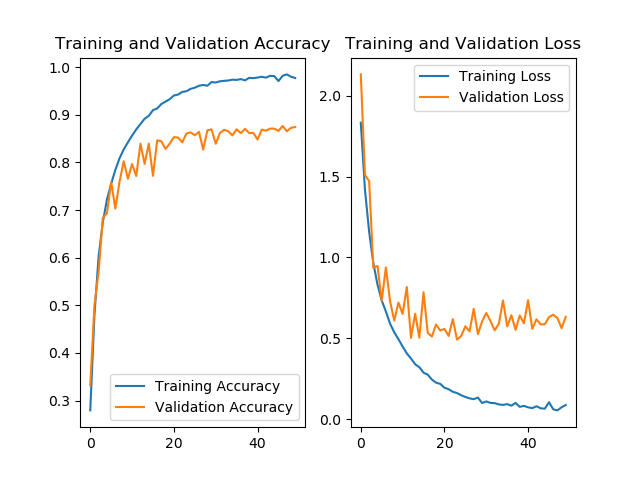Inception10：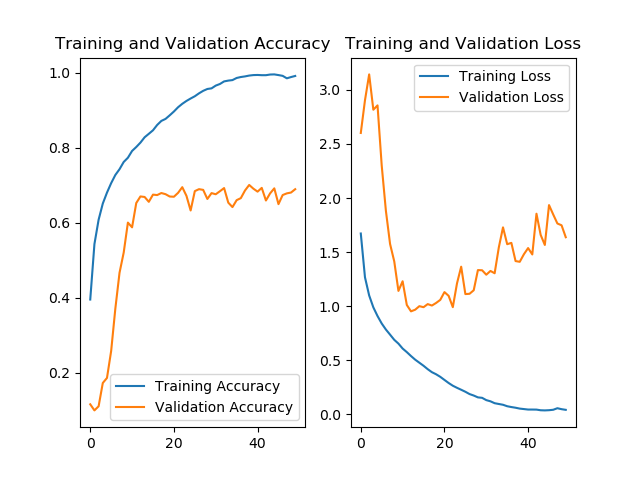ResNet18：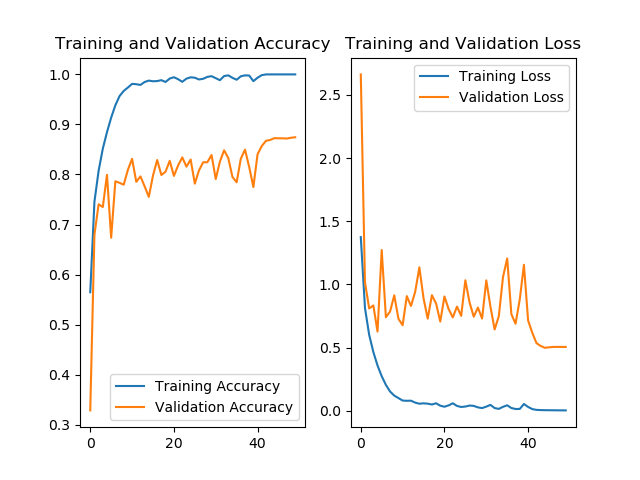### glut函数库_glut函数手册_hank_yan的博客-程序员秘密

GLUT函数说明 1一、初始化void glutInit(int* argc,char** argv)这个函数用来初始化GLUT库。对应main函数的形式应是：int main(int argc,char* argv[]); 这个函数从main函数获取其两个参数。void glutInitWindowSize(int width,int height);vo

### StringBuffer类的使用和详解底层实现、String和StringBuffer的区别、StringBuffer的常用方法_不等风雨，只等你的博客-程序员秘密

Java StringBuffer类的使用和详解1.java StringBuffer类2. StringBuffer类的构造器（构造方法）StringBuffer在工作的时候，使用的频率非常的高，常常会来拿来接收sql，并进行条件拼接。1.java StringBuffer类java.lang.StringBuffer代表可变的字符序列，可以对字符串内容进行增删。很多方法与String...